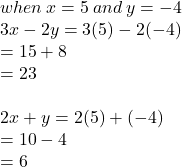## Explain or show that the point (5,−4) is a solution to this system of equations: 3x−2y=23 2x+y=6

Question

Explain or show that the point (5,−4) is a solution to this system of equations:

3x−2y=23

2x+y=6

in progress 0
2 months 2021-08-18T06:42:34+00:00 1 Answers 5 views 0